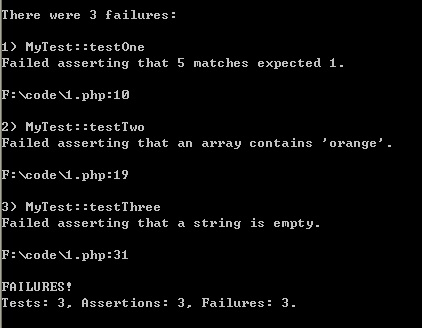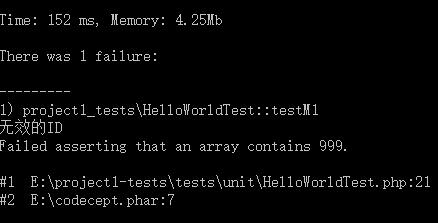# 单元测试 - 断言 ¶

• 作者：KK

• 发表日期：2015.12.13

## 提示：已经了解单元测试知道断言是咋回事的老鸟请直奔下一篇 ¶

``````public function testMe(){
\$this->assertTrue(1 + 2 == 3);	//第1次断言，等式成立，断言成功，继续往下运行
file_put_contents('E:\1.txt', 11);	//不信你看看，这个文件存在喔，说明这里被运行了
\$this->assertTrue(1 + 2 == 4);	//第2次断言，等式不成立，断言失败，下一句将无法运行，运行到这里就会退出这个测试方法，如果有其它测试方法就会再运行另一个测试方法
file_put_contents('E:\2.txt', 22);	//为了证明不会运行到这里，特设此代码，让你去找找2.txt是否真的不会被创建
\$this->assertTrue(2 + 2 == 4);	//第3次断言，等式成立，但上一句已经断言失败，这里不会被运行

//最终你就是能看到运行结果提示只运行了2次断言，1次成功1次失败，没有提到第3次断言，因为这个测试方法在第二句断言时就中断了，你也看不到 E:\2.txt 文件的存在
}
``````

## 断言的方式 ¶

``````\$this->assertTrue(is_string(\$name));	//断言名字是字符串来的
\$this->assertTrue(is_numerice(\$age));	//断言年龄是数值来的
\$this->assertTrue(is_numerice(\$age) && \$age >= 18);	//并且年龄还是18岁以上
\$this->assertTrue(\$action != '删除');	//断言一个操作标记不会是某个字符
\$this->assertTrue(mb_strlen(\$name) >= 6);	//断言一个名字是至少6个字的
\$this->assertTrue(\$xxx->getYYY() == \$qqq->ccc());	//断言两个方法的运行结果应该是相同的
\$this->assertTrue(isset(\$arr['xxx']));	//断言一个数组是有xxx这个下标的
``````

``````\$this->assertEquals(5, count(\$arr));	//断言一个数组count后的个数是否等于5
\$this->assertInstanceOf('app\model\User', \$user); //断言一个用户变量是 common\model\User 这个类的实例或子类实例
\$this->assertGreaterThan (10, \$age);		//断言年龄是大于10岁
\$this->assertContains(3, \$arr);	//断言数组里是包含了数字3这个值的
``````

## 不要老是使用assertTrue ¶``````\$id = 999;	//实际上可能是什么\$db->get('id')的代码赋值得来的嘛
\$this->assertTrue(in_array(\$id, [1, 2, 3]), '无效的ID');	//这里传了第2个参数，一个用于表达错误的消息字符串
``````